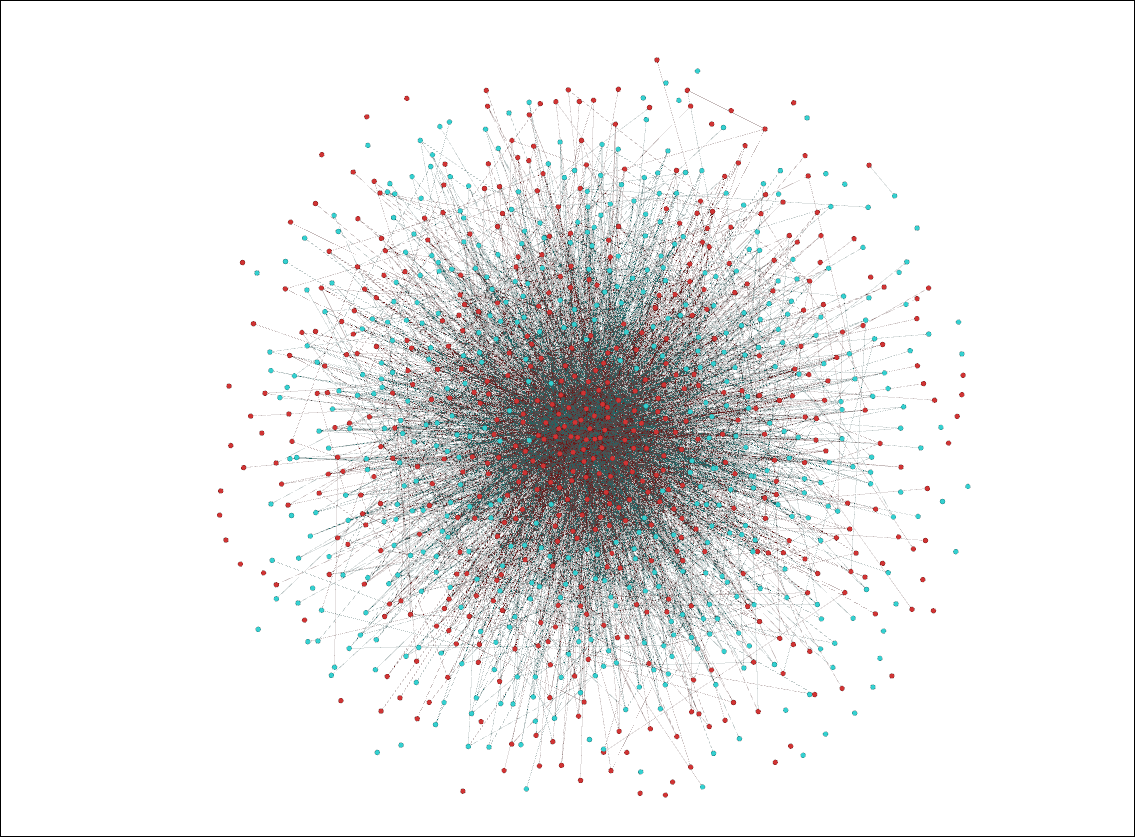hashkat.org

With the preferential_agent follow model, an agent follows first a preferred agent_type, then within that type shows preferential attachment to the agent with the highest number of followers (in-degree).

This simulation starts with the input file created in Tutorial 5. The input file that we create in this tutorial can be found for reference in /hashkat/docs/tutorial_input_files/tutorial06/INFILE.yaml. This tutorial should take approximately 10 minutes to complete.

#### Constructing The Network

Set:

• follow_model: preferential_agent

Luckily we already modified our input file for an agent follow model in the previous tutorial therefore all we have to do is change the follow model in INFILE.yaml to preferential_agent and #k@ will do the rest.

#### Running and Visualizing The Network

Running this simulation, we produced the following visualization:As you can see, not only do the 'Celebrity' agents (red nodes) have more connections than the 'Standard' agents (blue nodes), but with preferential attachment there are particular agents within each agent_type that have far more connections than other agents of the same type.

The cumulative-degree distribution for this network is shown below:As we can see, the majority of agents in this network have a combined number of followers & following (cumulative degree) less than 20, while a few agents have cumulative degrees up to almost 180.

The commands used to plot this graph in Gnuplot were:

`set style fill transparent solid 0.5 noborder`

`set title 'Cumulative-Degree Distribution'`

`set xlabel 'Cumulative-Degree'`

`set ylabel 'Normalized Cumulative-Degree Probability'`

`plot 'Celebrity_info.dat' u 1:4 lc rgb 'goldenrod' w filledcurves above y1=0 title 'Celebrity',` `'Standard_info.dat' u 1:4 lc rgb 'blue' w filledcurves above y1=0 title 'Standard',` `'cumulative-degree_distribution_month_000.dat' u 1:2 lc rgb 'dark-red' w filledcurves above y1=0` `title 'Overall'`

#### Next Steps

You have now familiarity with the preferential_agent follow model. In the next tutorial, where we demonstrate the hashtag follow model.